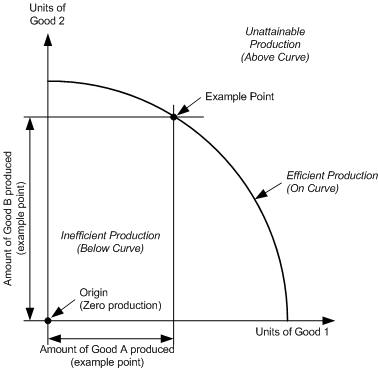## Quiz 1 : Alleviating Human MiseryLooking for Economics Homework Help?# Quiz 1 : Alleviating Human Misery

Gross domestic product (GDP): Gross domestc product (GDP) is defind as the total market value of all the final goods and services produced within the physical boundary of a nation at a particular time period. GDP as a measure of social well-being: There are mainly two adjustments of GDP that are required before it can be employed as a rough measure of social well-being. They are listed below: Adjusting GDP for inflation: Sometimes, GDP may be doubled, even though the production of goods remains the same. This change in GDP is due to inflation. To remove the effect of inflation each year, the price levels of GDP are replaced with the price of the 'base' year. In percentage terms, the price index for each year is derived by dividing each year's price level by the base year's price level and multiplying it with 100. Adjusting GDP for population: The adjustment of GDP made for dissimilarities in population requires that GDP should be divided by the population of the country, that is, the per capita GDP. This method gives a rough quantification of its citizen's average well-being.

The gross domestic product (GDP) represents the market value of all the goods and services produced in a country, usually for a given year, and typically expressed in dollars. Because GDP is calculated using the current market prices belonging to the year that the measurement is made, increases in market prices will also increase the GDP, even if the economy did not actually grow. Therefore, an increase in GDP does not necessarily mean that the economy of the country increased. High inflation could increase GDP even when the economy shrinks. To account for the effects of changes in market prices, the GDP can be adjusted using a price index. The price index for a given year represents the relative cost of goods and services relative to other years. For example, if the price index for year A iS₁00 and the price index for year B iS₁10, then prices in year B were 110% of the prices in year B. Usually a certain year is selected as the reference year to express GDP values in. The price index for the reference year is set to 100, and the price indices for other years are scaled proportionately. Multiplying GDP by the price index (in percent) causes GDP to be expressed in terms of dollars in the reference year. This is referred to as real GDP. Therefore, GDP is the value of goods and services in current-year market prices, while real GDP is the value of goods in service in reference-year market prices.

The production possibilities curve illustrates conceptually how resources in an economy can be divided between competing goods or services. The plot shows the potential amounts of production of two competing goods. An example of a production possibilities curve is shown below.Any given point in the graph represents a possible combination of amounts of good 1 and good 2. The horizontal distance of the point from the origin represents the amount of production of good 1 while the vertical distance represents the amount of production of good 2. The curve itself defines the region of production that the economy is capable of achieving. Points at or below the curve are within the capability of the economy, while points above the curve represent production levels that cannot be attained. Points on the curve itself represent efficient production, because the output of the economy is maximized. Points below the curve represent inefficient production, because the output of either good could be increased without decreasing the production of the other. The idea of opportunity cost is represented by points along the line. That is, given that the economy cannot move production outside of the region defined by the curve, then efficient production can only achieve combinations of good 1 and good 2 represented by points along the curve. Therefore, an increase or decrease in production of good 1 comes with a corresponding decrease or increase in production of good 2. In other words, any increase in production of either good comes at the opportunity cost of a reduction in the other good, when the economy is operating efficiently.